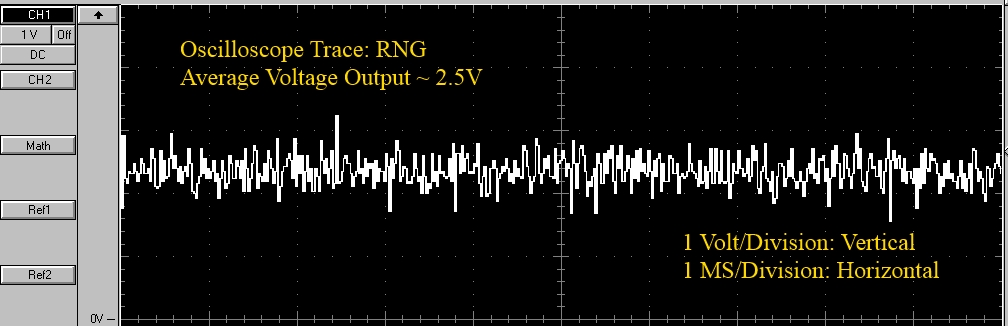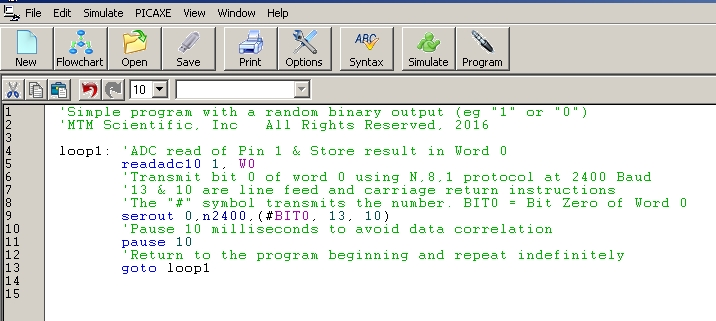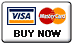# Build a Hardware Random Number Generator

## by MTM Scientific, Inc.

``

## Figure 1. Assembled circuit of the hardware random number generator.

This simple hardware Random Number Generator (RNG) project is designed to create a continuous string of completely random numbers. The numbers are generated from the random noise of a reverse-biased semiconductor junction in a transistor. The basic unit of numerical output from the RNG is a continuous string of random bits (e.g. "1" or "0"). The individual bits can be combined to create any other type of output... such as numbers, text strings, passwords or ASCII symbols.

The data output from the RNG is a standard serial data stream. The output can be collected and recorded by any computer with a serial port. The output from this RNG is random, but not especially fast. This RNG design is presented for hobby purposes. We make no warranty about the suitability of the output for applications requiring perfectly random numbers. Figure 1 shows the assembled RNG and Figure 2 shows the electrical schematic.

``

## Figure 2. Electrical circuit of the hardware random number generator.

Generating truly random numbers is surprisingly difficult to do. Stated another way, generating numbers without any traces of a repeating or predictable pattern is very challenging. Computers are notoriously bad at generating random numbers. Which makes sense: Computers predictably perform the instructions they are given to generate numerical output. Hardware Random Number Generators take a different approach to generating random numbers. The RNG begins with a physical process that is intrinsically random. The physical output of the random process is then used to generate the random numbers.

In this RNG the random physical process is the electrical noise generated in the reverse-biased semiconductor junction of a 2N3904 transistor. The 12V reverse bias was carefully chosen to generate sufficient noise, but not to damage the transistor. The noise from the transistor is amplified by a second transistor. The signal from the second transistor is capacitively coupled to a resistive voltage divider. The result is an analog noise signal approximately centered at a level of 2.5V as shown in the oscilloscope trace in Figure 3.

``

## Figure 3. Oscilloscope trace of the analog output from the transistor noise generator.

The noise voltage from the transistor circuit is converted to a number using an analog to digital converter. After conversion, the numerical result is serially transmitted to a host computer. The conversion and transmission are both easily implemented by using a PICAXE-08M2 microcontroller. The PICAXE-08M2 is essentially a small computer on a chip. The PICAXE is programmed using a simple computer language called 'Picaxe BASIC'. A sample program listing is shown in Figure 4.

``

## Figure 4. Picaxe Basic program to read and send serial data output.

The analog to digital (ADC) conversion generates a binary number 10 bits wide. But not all 10 bits have the same degree of randomness, as shown in Figure 5. We chose to only use the least significant bit of the conversion. The least significant bit represents a voltage change of only about 5 millivolts (0.005 Volts). The bit with the lowest amount of randomness is the most significant bit of the ADC conversion, which makes sense. In the program listing you will notice that a time delay was introduced between ADC readings. This was done to prevent correlation between consecutive readings.

``

## Figure 5. Histogram analysis of RNG bit level output.

The string of bits from the ADC conversion can be combined to create other types of random output. For example, by combining 8 bits together we can create a byte (Numerical range 0-255). A byte can also represent an ASCII character, per the standard encoding table. The data output conversions can be programmed using simple Picaxe BASIC commands in the PICAXE microcontroller, before the data is serially transmitted. The randomness of the output can be tested using special tests created for the purpose. For example, the 'DIEHARD' suite of tests written by George Marsaglia.``